# Rational Functions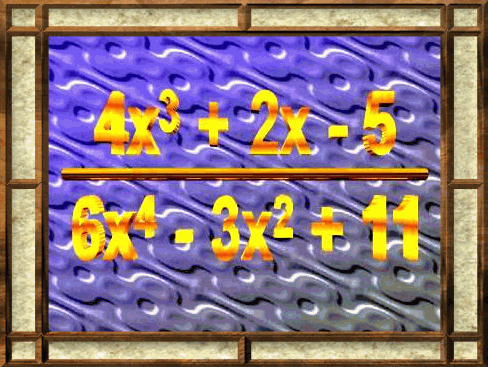A rational function is formed when a polynomial is divided by another polynomial. Below are some links to sections dealing with rational functions. There is a wealth of material here that would be helpful to any student of mathematics studying this topic.

The following link takes you to an overview of rational functions. It presents a definition of a rational function as long as some introductory material concerned with finding the roots and discontinuities of rational functions.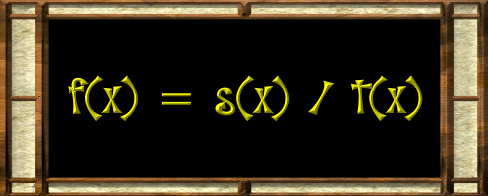These next links present some fundamental ideas concerning one of the simplest of rational functions, f(x) = 1/x.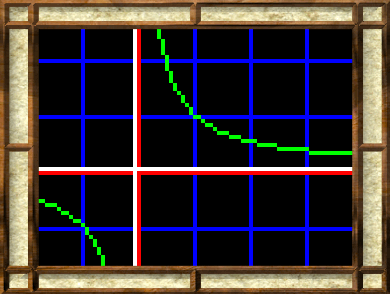This next link gives a detailed explanation of how to work with a rational function. This includes a complete presentation of how to find roots, discontinuities, and end behavior.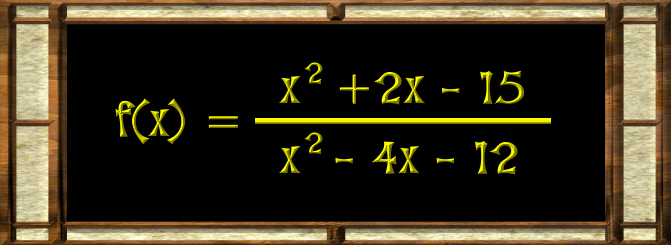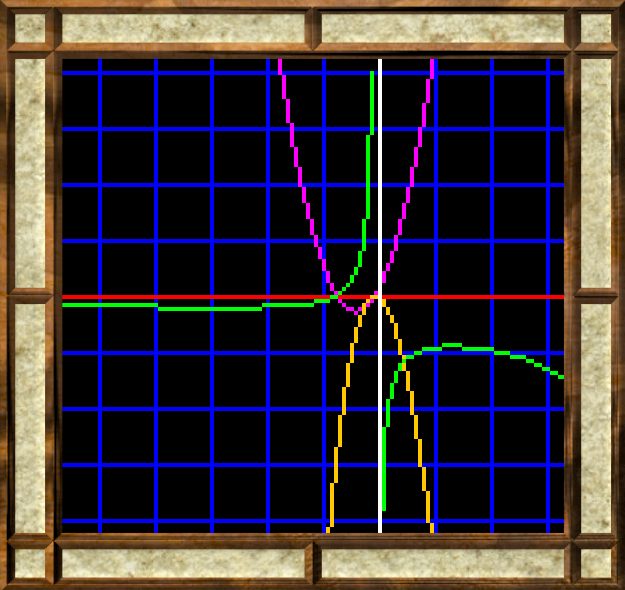Custom Search